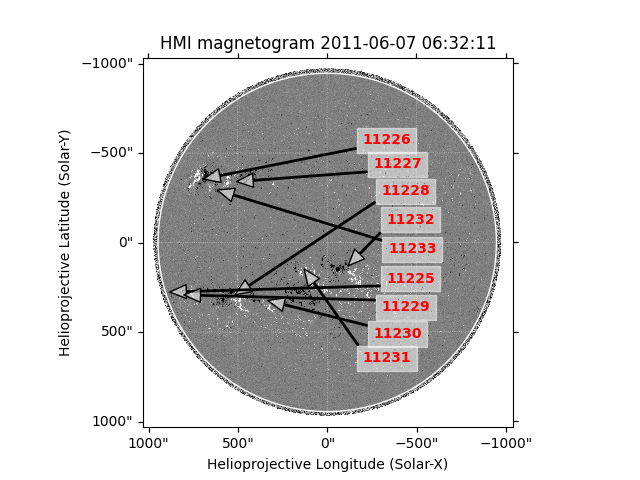# Overplotting SRS active region locations on a magnetograms¶

How to find and plot the location of an active region on an HMI magnetogram.

```import matplotlib.pyplot as plt
import numpy as np

import sunpy.coordinates
import sunpy.data.sample
import sunpy.map
from sunpy.io.special import srs
```

For this example, we will start with the sample data. We need an HMI file and use it to create a map, and the SRS table which contains a list of active regions. Both of these data can be downloaded with `Fido`.

```smap = sunpy.map.Map(sunpy.data.sample.HMI_LOS_IMAGE)
```

We only need the rows which have ‘ID’ = ‘I’ or ‘IA’.

```srs_table = srs_table[np.logical_or(srs_table['ID'] == 'I', srs_table['ID'] == 'IA')]
```

Now we extract the latitudes, longitudes and the region numbers. `plot_coord()` will error on some versions of Astropy if a coordinate component contains a mask, but since none of the masks in these arrays here actually mask any elements, we simply remove the masks.

```lats = srs_table['Latitude']
lngs = srs_table['Longitude']
numbers = srs_table['Number']
```

Let’s plot the results by defining coordinates for each location.

```fig = plt.figure()
# Passing vmin/vmax to ``plot`` does not work since
# a normalisation is set on the map. So we have to
# work around it like so:
smap.plot_settings["norm"].vmin = -150
smap.plot_settings["norm"].vmax = 150
smap.plot(axes=ax)
smap.draw_limb(axes=ax)

# Add a text box and arrow pointing to each active region
lat_text = -40
transparent_white = (1, 1, 1, 0.5)
for num, lng, lat in zip(numbers, lngs.value, lats.value):
ax.annotate(num, (lng, lat),
xytext=(320, lat_text),
xycoords=ax.get_transform('heliographic_stonyhurst'),
backgroundcolor=transparent_white,
color='red',
fontweight='bold',